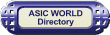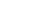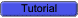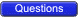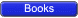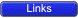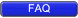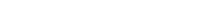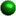Error Detecting and Correction Codes For reliable transmission and storage of digital data, error detection and correction is required. Below are a few examples of codes which permit error detection and error correction after detection.Error Detecting Codes When data is transmitted from one point to another, like in wireless transmission, or it is just stored, like in hard disks and memories, there are chances that data may get corrupted. To detect these data errors, we use special codes, which are error detection codes.Parity In parity codes, every data byte, or nibble (according to how user wants to use it) is checked if they have even number of ones or even number of zeros. Based on this information an additional bit is appended to the original data. Thus if we consider 8-bit data, adding the parity bit will make it 9 bit long.At the receiver side, once again parity is calculated and matched with the received parity (bit 9), and if they match, data is ok, otherwise data is corrupt.There are two types of parity: Even parity: Checks if there is an even number of ones; if so, parity bit is zero. When the number of ones is odd then parity bit is set to 1. Odd Parity: Checks if there is an odd number of ones; if so, parity bit is zero. When number of ones is even then parity bit is set to 1.Check Sums The parity method is calculated over byte, word or double word. But when errors need to be checked over 128 bytes or more (basically blocks of data), then calculating parity is not the right way. So we have checksum, which allows to check for errors on block of data. There are many variations of checksum.Adding all bytes CRC Fletcher's checksum Adler-32The simplest form of checksum, which simply adds up the asserted bits in the data, cannot detect a number of types of errors. In particular, such a checksum is not changed by:Reordering of the bytes in the message Inserting or deleting zero-valued bytes Multiple errors which sum to zeroExample of Checksum : Given 4 bytes of data (can be done with any number of bytes): 25h, 62h, 3Fh, 52hAdding all bytes together gives 118h. Drop the Carry Nibble to give you 18h. Get the two's complement of the 18h to get E8h. This is the checksum byte.To Test the Checksum byte simply add it to the original group of bytes. This should give you 200h. Drop the carry nibble again giving 00h. Since it is 00h this means the checksum means the bytes were probably not changed.Error-Correcting Codes Error-correcting codes not only detect errors, but also correct them. This is used normally in Satellite communication, where turn-around delay is very high as is the probability of data getting corrupt.ECC (Error correcting codes) are used also in memories, networking, Hard disk, CDROM, DVD etc. Normally in networking chips (ASIC), we have 2 Error detection bits and 1 Error correction bit.Hamming Code Hamming code adds a minimum number of bits to the data transmitted in a noisy channel, to be able to correct every possible one-bit error. It can detect (not correct) two-bits errors and cannot distinguish between 1-bit and 2-bits inconsistencies. It can't - in general - detect 3(or more)-bits errors.The idea is that the failed bit position in an n-bit string (which we'll call X) can be represented in binary with log2(n) bits, hence we'll try to get it adding just log2(n) bits.First, we set m = n + log2(n) to the encoded string length and we number each bit position starting from 1 through m. Then we place these additional bits at power-of-two positions, that is 1, 2, 4, 8..., while remaining ones (3, 5, 6, 7...) hold the bit string in the original order.Now we set each added bit to the parity of a group of bits. We group bits this way: we form a group for every parity bit, where the following relation holds:position(bit) AND position(parity) = position(parity) (Note that: AND is the bit-wise boolean AND; parity bits are included in the groups; each bit can belong to one or more groups.)So bit 1 groups bits 1, 3, 5, 7... while bit 2 groups bits 2, 3, 6, 7, 10... , bit 4 groups bits 4, 5, 6, 7, 12, 13... and so on.Thus, by definition, X (the failed bit position defined above) is the sum of the incorrect parity bits positions (0 for no errors).To understand why it is so, let's call Xn the nth bit of X in binary representation. Now consider that each parity bit is tied to a bit of X: parity1 -> X1, parity2 -> X2, parity4 -> X3, parity8 -> X4 and so on - for programmers: they are the respective AND masks -. By construction, the failed bit makes fail only the parity bits which correspond to the 1s in X, so each bit of X is 1 if the corresponding parity is wrong and 0 if it is correct.Note that the longer the string, the higher the throughput n/m and the lower the probability that no more than one bit fails. So the string to be sent should be broken into blocks whose length depends on the transmission channel quality (the cleaner the channel, the bigger the block). Also, unless it's guaranteed that at most one bit per block fails, a checksum or some other form of data integrity check should be added.Alphanumeric Codes The binary codes that can be used to represent all the letters of the alphabet, numbers and mathematical symbols, punctuation marks, are known as alphanumeric codes or character codes. These codes enable us to interface the input-output devices like the keyboard, printers, video displays with the computer.ASCII Code ASCII stands for American Standard Code for Information Interchange. It has become a world standard alphanumeric code for microcomputers and computers. It is a 7-bit code representing 27 = 128 different characters. These characters represent 26 upper case letters (A to Z), 26 lowercase letters (a to z), 10 numbers (0 to 9), 33 special characters and symbols and 33 control characters.The 7-bit code is divided into two portions, The leftmost 3 bits portion is called zone bits and the 4-bit portion on the right is called numeric bits.An 8-bit version of ASCII code is known as USACC-II 8 or ASCII-8. The 8-bit version can represent a maximum of 256 characters.EBCDIC Code EBCDIC stands for Extended Binary Coded Decimal Interchange. It is mainly used with large computer systems like mainframes. EBCDIC is an 8-bit code and thus accomodates up to 256 characters. An EBCDIC code is divided into two portions: 4 zone bits (on the left) and 4 numeric bits (on the right).